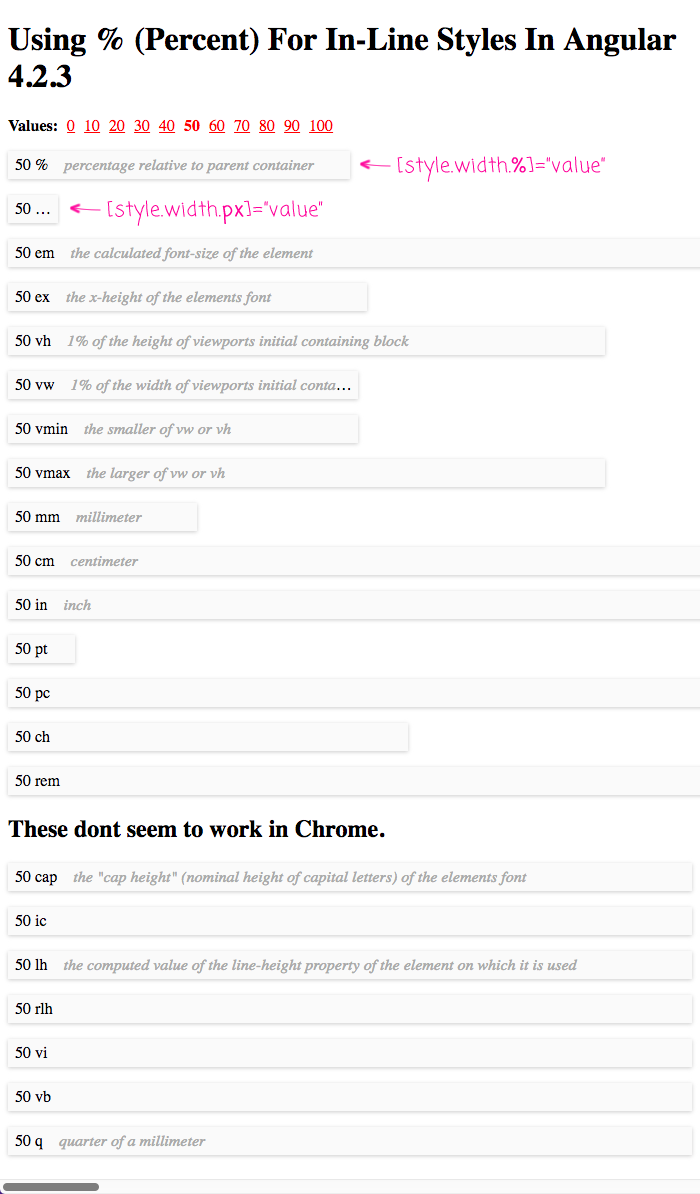On User Experience (UX) Design, JavaScript, ColdFusion, Node.js, Life, and Love.

# Using % (Percent) For In-Line Styles In Angular 4.2.3

By on

This is a super minor post; but, I just used the "%" (percent) symbol for in-line styles in Angular 4.2.3, and it totally worked. It's just another example of how the Angular template syntax is such a joy to work with. Little things like CSS unit-selection become friction-free tasks. No more messing around with string interpolation or string concatenation - just select the unit of measurement and apply the length value.

This is barely worth sharing, since this functionality is all in the Angular documentation. But, the Angular template syntax makes me so happy, I can't help but want to be a share-bear. When defining an in-line style, the unit of measurement can be provided as part of the attribute name. Here, we're using the "px" - pixel - unit:

<p [style.width.px]="someValue"> ... </p>

It's that easy! And here, we're using the "%" - percent - unit:

<p [style.width.%]="someValue"> ... </p>

Doesn't that just make you smile? To see this in action, I've created a small demo in which I use a variety of CSS units to render the same numeric value:

• // Import the core angular services.
• import { Component } from "@angular/core";
•
• @Component({
• selector: "my-app",
• styleUrls: [ "./app.component.css" ],
• template:
• `
• <p class="actions">
• <strong>Values:</strong>
•
• <ng-template ngFor let-i [ngForOf]="[ 0, 10, 20, 30, 40, 50, 60, 70, 80, 90, 100 ]">
•
• <a (click)="setValue( i )" [class.selected]="( i === value )">
• {{ i }}
• </a>
•
• </ng-template>
• </p>
•
• <p class="bar" [style.width.%]="value">
• {{ value }} % <span>percentage relative to parent container</span>
• </p>
•
• <p class="bar" [style.width.px]="value">
• {{ value }} px <span>absolute pixel</span>
• </p>
•
• <p class="bar" [style.width.em]="value">
• {{ value }} em <span>the calculated font-size of the element</span>
• </p>
•
• <p class="bar" [style.width.ex]="value">
• {{ value }} ex <span>the x-height of the elements font</span>
• </p>
•
• <p class="bar" [style.width.vh]="value">
• {{ value }} vh <span>1% of the height of viewports initial containing block</span>
• </p>
•
• <p class="bar" [style.width.vw]="value">
• {{ value }} vw <span>1% of the width of viewports initial containing block</span>
• </p>
•
• <p class="bar" [style.width.vmin]="value">
• {{ value }} vmin <span>the smaller of vw or vh</span>
• </p>
•
• <p class="bar" [style.width.vmax]="value">
• {{ value }} vmax <span>the larger of vw or vh</span>
• </p>
•
• <p class="bar" [style.width.mm]="value">
• {{ value }} mm <span>millimeter</span>
• </p>
•
• <p class="bar" [style.width.cm]="value">
• {{ value }} cm <span>centimeter</span>
• </p>
•
• <p class="bar" [style.width.in]="value">
• {{ value }} in <span>inch</span>
• </p>
•
• <p class="bar" [style.width.pt]="value">
• {{ value }} pt
• </p>
•
• <p class="bar" [style.width.pc]="value">
• {{ value }} pc
• </p>
•
• <p class="bar" [style.width.ch]="value">
• {{ value }} ch
• </p>
•
• <p class="bar" [style.width.rem]="value">
• {{ value }} rem
• </p>
•
• <h2>
• These dont seem to work in Chrome.
• </h2>
•
• <p class="bar" [style.width.cap]="value">
• {{ value }} cap <span>the "cap height" (nominal height of capital letters) of the elements font</span>
• </p>
•
• <p class="bar" [style.width.ic]="value">
• {{ value }} ic
• </p>
•
• <p class="bar" [style.width.lh]="value">
• {{ value }} lh <span>the computed value of the line-height property of the element on which it is used</span>
• </p>
•
• <p class="bar" [style.width.rlh]="value">
• {{ value }} rlh
• </p>
•
• <p class="bar" [style.width.vi]="value">
• {{ value }} vi
• </p>
•
• <p class="bar" [style.width.vb]="value">
• {{ value }} vb
• </p>
•
• <p class="bar" [style.width.q]="value">
• {{ value }} q <span>quarter of a millimeter</span>
• </p>
• `
• })
• export class AppComponent {
•
• public value: number;
•
•
• // I initialize the app component.
• constructor() {
•
• this.value = 50;
•
• }
•
•
• // ---
• // PUBLIC METHODS.
• // ---
•
•
• // I set the value used to display the various dimensional units.
• public setValue( value: number ) : void {
•
• this.value = value;
•
• }
•
• }

As you can see, each P tag is rendering the same "value" component property. But, each P tag uses a different unit of measurement for its in-line style. And, when we run this code, you can see how each unit affects the rendering:So cool!

When you first see the Angular template syntax, it's a little jaring. Kind of like the first time you see JSX in ReactJS. But, once you start using it, the Angular template syntax just brings joy. Being able to use CSS units, like "%", with your in-line styles is just one example of this holistic design.You know what, it may be in the documentation, but I hadn't seen it used like that before. That is pretty cool that you can straight up use .% and it works as you would expect it to. There's a lot of little things I feel like they got right the second time around with Angular. And those "little things" make a difference.@John,

Agreed! And, as much documentation as there is, little things are not always well articulated. For example, the documentation doesn't ever explicitly say that you can use "%". Instead, it just says you can use CSS units. So, it takes some mental gymnastics to get from that statement to actually trying to see if "%" works :)

That said, I really do like the Angular 2 template syntax. Much to like.Thanks Ben, one more time you save me a lot of time :)Thank you !Glad you are all liking this -- it's so easy, I love it!